1. Worksheets>
2. Math>
3. Geometry>
4. Distance Formula

Distance Formula Worksheets

This module helps find the distance between two points (x1, y1) and (x2, y2). It consists of worksheets to find the length of the line segments. It also includes worksheets based on the classification of triangles and quadrilaterals. Besides, application of the distance formula in geometry is included here.

Length of a line segment: Number line

Find the length of the line segments in each number line. Write the answer in units. Each worksheet consists of three number lines.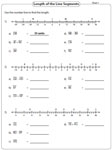Distance Formula: Graph

A line segment is drawn on each grid. Find the length of the line segment. Round the answer to two decimal places.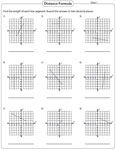Distance Formula: Standard

Using the distance formula, find the distance between two points. There are ten problems in each worksheet.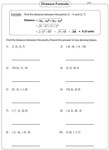Level 1:

Level 2:

Length of the side: Shapes

Apply the distance formula to determine the length of the side in each shape. Round the answer to two decimal places.Length of a line segment: Graph

Using the distance formula, find the length of the line segments (horizontal, vertical and diagonal).Finding a missing coordinate

In these worksheets, find the missing coordinate with the given endpoints and the distance between them.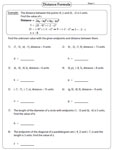Distance formula and Pythagorean theorem

Find the length of the line segments using the Pythagorean theorem. Use the distance formula to verify the answer.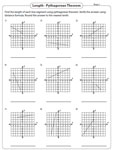Identify the type - Triangles

The vertices of the triangle are given in each problem. Apply the distance formula to prove whether the triangle is a scalene, equilateral, isosceles or right triangle.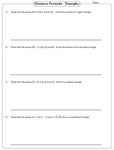Identify the type - Quadrilaterals

The vertices of the quadrilateral are given in each problem. Apply the distance formula to prove whether the quadrilateral is a rhombus, square, rectangle or parallelogram.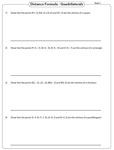Distance formula - Perimeter

Plot the points on the grid. Apply the formula to find the perimeter of each shape.Midpoint and Distance formula

Apply mid-point formula and distance formula to find the missing length of the given geometrical figures.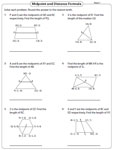Applications in geometry

These worksheets comprise of various geometrical figures. Find the missing length. Round the answer to the nearest tenth.Stock up your library with the complete set of worksheets on distance formula.

Related Worksheets